# Logarithmic scoring rule is proper

## Statement

The logarithmic scoring rule is a proper scoring rule. Explicitly:

Consider a random variable$X$ that can take$n$ distinct values$1,2,\dots,n$. Suppose we estimate probabilities$p_1,p_2,\dots,p_n$ for these values respectively (with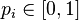$p_i \in [0,1]$,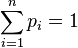$\sum_{i=1}^n p_i = 1$). The logarithmic scoring rule works as follows: for every instance of the random variable$X$, we assign score equal to the negative of the logarithm of the corresponding probability$p_i$. Explicitly, if the instances are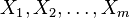$X_1,X_2,\dots,X_m$, the total score is: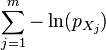$\sum_{j=1}^m -\ln(p_{X_j})$

The claim is that, if the actual probabilities are$q_1,q_2,\dots,q_n$, then the assignment that minimizes the expected value of the score is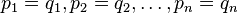$p_1 = q_1, p_2 = q_2,\dots, p_n = q_n$.

## Proof

### Reduction to one random instance

Under the assumption that the instances are independent of each other, it suffices to show the result for one instance.

### Reduction to the case that all probabilities are strictly between zero and one

We now show that if any particular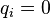$q_i = 0$, the corresponding$p_i$ must equal 0, and if any$q_i = 1$, the corresponding$p_i$ must equal 1.

Fill this in later

### Proof for one instance and where all the actual probabilities are nonzero and less than one

The expected value for one instance is:$\sum_{i=1}^n -q_i \ln (p_i)$

In words, we weight each score by the probability that that score is attained.

We are constrained to lie on the codimension one hyperplane given by$\sum_{i=1}^n p_i = 1$. We can therefore use the idea of Lagrange multipliers to find the optima. The gradient vector of the expected value function is the vector with coordinates: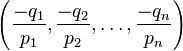$\left(\frac{-q_1}{p_1},\frac{-q_2}{p_2},\dots,\frac{-q_n}{p_n}\right)$

The normal vector to the hyperplane is given as the gradient vector of the function$\sum_{i=1}^n p_i$, and is the vector: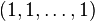$(1,1,\dots,1)$

By the theory of Lagrange multipliers, we have that at any local extreme value, there exists a value$\lambda$ such that:$\frac{-q_i}{p_i} = \lambda(1)$

for all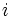$i$. In other words: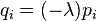$q_i = (-\lambda)p_i$

for all$i$. Adding up, we get:$\sum_{i=1}^n q_i = (-\lambda) \sum_{i=1}^n p_i$

We have that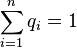$\sum_{i=1}^n q_i = 1$ as well (these are the actual probabilities, so they add up to 1), so we get: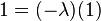$1 = (-\lambda)(1)$

so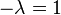$-\lambda = 1$. Plugging back, we get that the only point that could potentially be a point of local extremum satisfies$q_i = p_i$ for all$i$.

We can now verify that this is indeed a point of local minimum. Fill this in later

We can also verify that the absolute minimum does not occur at the boundary: if$q_i \ne 0$ but we set$p_i = 0$, then our expected score is$\infty$, because there's a nonzero probability of paying an infinite cost, namely, in the case that the random variable takes the value$i$.